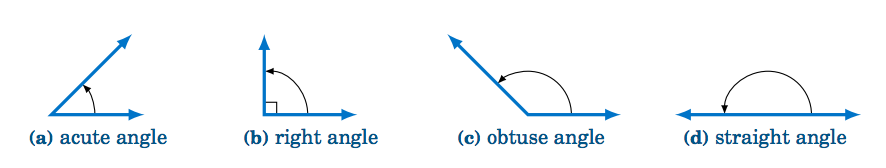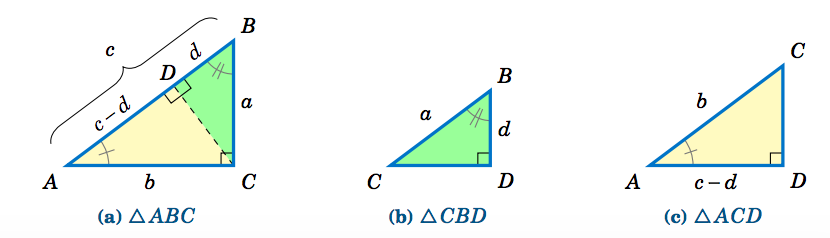$$\newcommand{\id}{\mathrm{id}}$$ $$\newcommand{\Span}{\mathrm{span}}$$ $$\newcommand{\kernel}{\mathrm{null}\,}$$ $$\newcommand{\range}{\mathrm{range}\,}$$ $$\newcommand{\RealPart}{\mathrm{Re}}$$ $$\newcommand{\ImaginaryPart}{\mathrm{Im}}$$ $$\newcommand{\Argument}{\mathrm{Arg}}$$ $$\newcommand{\norm}{\| #1 \|}$$ $$\newcommand{\inner}{\langle #1, #2 \rangle}$$ $$\newcommand{\Span}{\mathrm{span}}$$

# 1.1: Angles

•• Michael Corral
• Professor (Mathematics) at Schoolcraft College
$$\newcommand{\vecs}{\overset { \rightharpoonup} {\mathbf{#1}} }$$ $$\newcommand{\vecd}{\overset{-\!-\!\rightharpoonup}{\vphantom{a}\smash {#1}}}$$$$\newcommand{\id}{\mathrm{id}}$$ $$\newcommand{\Span}{\mathrm{span}}$$ $$\newcommand{\kernel}{\mathrm{null}\,}$$ $$\newcommand{\range}{\mathrm{range}\,}$$ $$\newcommand{\RealPart}{\mathrm{Re}}$$ $$\newcommand{\ImaginaryPart}{\mathrm{Im}}$$ $$\newcommand{\Argument}{\mathrm{Arg}}$$ $$\newcommand{\norm}{\| #1 \|}$$ $$\newcommand{\inner}{\langle #1, #2 \rangle}$$ $$\newcommand{\Span}{\mathrm{span}}$$ $$\newcommand{\id}{\mathrm{id}}$$ $$\newcommand{\Span}{\mathrm{span}}$$ $$\newcommand{\kernel}{\mathrm{null}\,}$$ $$\newcommand{\range}{\mathrm{range}\,}$$ $$\newcommand{\RealPart}{\mathrm{Re}}$$ $$\newcommand{\ImaginaryPart}{\mathrm{Im}}$$ $$\newcommand{\Argument}{\mathrm{Arg}}$$ $$\newcommand{\norm}{\| #1 \|}$$ $$\newcommand{\inner}{\langle #1, #2 \rangle}$$ $$\newcommand{\Span}{\mathrm{span}}$$

Recall the following definitions from elementary geometry:

1. An angle is acute if it is between $$0°$$ and $$90°$$.
2. An angle is a right angle if it equals $$90°$$.
3. An angle is obtuse if it is between $$90°$$ and $$180°$$.
4. An angle is a straight angle if it equals $$180°$$.Figure 1.1.1 Types of angles

In elementary geometry, angles are always considered to be positive and not larger than $$360^\circ$$. For now we will only consider such angles. The following definitions will be used throughout the text:

1. Two acute angles are complementary if their sum equals $$90^◦$$. In other words, if $$0^◦ ≤ ∠ A , ∠B ≤ 90^◦ \text{ then }∠ A \text{ and }∠B$$ are complementary if $$∠ A +∠B = 90^◦$$.
2. Two angles between $$0^◦ \text{ and }180^◦$$ are supplementary if their sum equals $$180^◦$$. In other words, if $$0^◦ ≤ ∠ A , ∠B ≤ 180^◦ \text{ then }∠ A \text{ and }∠B$$ are supplementary if $$∠ A +∠B = 180^◦$$.
3. Two angles between $$0^◦ \text{ and }360^◦$$ are conjugate (or explementary) if their sum equals $$360^◦$$. In other words, if $$0^◦ ≤ ∠ A , ∠B ≤ 360^◦ \text{ then }∠ A \text{ and }∠B\text{ are conjugate if }∠ A+∠B = 360^◦$$.Figure 1.1.2 Types of pairs of angles

Instead of using the angle notation $$∠ A$$ to denote an angle, we will sometimes use just a capital letter by itself (e.g. $$A, B, C$$) or a lowercase variable name (e.g. $$x, y, t$$). It is also common to use letters (either uppercase or lowercase) from the Greek alphabet, shown in the table below, to represent angles:

Table 1.1 The Greek alphabetIn elementary geometry you learned that the sum of the angles in a triangle equals $$180^◦$$, and that an isosceles triangle is a triangle with two sides of equal length. Recall that in a right triangle one of the angles is a right angle. Thus, in a right triangle one of the angles is $$90^◦$$ and the other two angles are acute angles whose sum is $$90^◦$$ (i.e. the other two angles are complementary angles).

$\nonumber α + 3α + α = 180^◦ ⇒ 5α = 180^◦ ⇒ α = 36^◦ ⇒ \fbox{$$X = 36^◦ ,\, Y = 3×36^◦ = 108^◦ ,\, Z = 36^◦$$}$

QED

By knowing the lengths of two sides of a right triangle, the length of the third side can be determined by using the Pythagorean Theorem:

The square of the length of the hypotenuse of a right triangle is equal to the sum of the squares of the lengths of its legs.Figure 1.1.5 Similar triangles $$△ ABC, △CBD, △ ACD$$

Recall that triangles are similar if their corresponding angles are equal, and that similarity implies that corresponding sides are proportional. Thus, since $$\triangle\,ABC$$ is similar to $$\triangle\,CBD$$, by proportionality of corresponding sides we see that

$\nonumber \overline{AB}~\text{is to}~\overline{CB}~\text{(hypotenuses)}\text{ as } \overline{BC}~\text{is to}~\overline{BD}~\text{(vertical legs)} \quad\Rightarrow\quad \frac{c}{a} ~=~ \frac{a}{d} \quad\Rightarrow\quad cd ~=~ a^2 ~.$

Since $$\triangle\,ABC$$ is similar to $$\triangle\,ACD$$, comparing horizontal legs and hypotenuses gives

$\nonumber \frac{b}{c-d} ~=~ \frac{c}{b} \quad\Rightarrow\quad b^2 ~=~ c^2 ~-~ cd ~=~ c ^2 ~-~ a^2 \quad\Rightarrow\quad a^2 ~+~ b^2 ~=~ c^2 ~. \textbf{QED}$

Note: The symbols $$\perp$$ and $$\sim$$ denote perpendicularity and similarity, respectively. For example, in the above proof we had $$\,\overline{CD} \perp \overline{AB}\,$$ and $$\,\triangle\,ABC \sim \triangle\,CBD \sim \triangle\,ACD$$.

For triangle $$\triangle\,ABC$$, the Pythagorean Theorem says that

$\nonumber a^2 ~+~ 4^2 ~=~ 5^2 \quad\Rightarrow\quad a^2 ~=~ 25 ~-~ 16 ~=~ 9 \quad\Rightarrow\quad \fbox{$$a ~=~ 3$$} ~.$

For triangle $$\triangle\,DEF$$, the Pythagorean Theorem says that

$\nonumber e^2 ~+~ 1^2 ~=~ 2^2 \quad\Rightarrow\quad e^2 ~=~ 4 ~-~ 1 ~=~ 3 \quad\Rightarrow\quad \fbox{e ~=~ \sqrt{3}} ~.$

For triangle $$\triangle\,XYZ$$, the Pythagorean Theorem says that

$\nonumber 1^2 ~+~ 1^2 ~=~ z^2 \quad\Rightarrow\quad z^2 ~=~ 2 \quad\Rightarrow\quad \fbox{z ~=~ \sqrt{2}} ~.$

Let $$h$$ be the height at which the ladder touches the wall. We can assume that the ground makes a right angle with the wall, as in the picture on the right. Then we see that the ladder, ground, and wall form a right triangle with a hypotenuse of length 17 ft (the length of the ladder) and legs with lengths 8 ft and $$h$$ ft. So by the Pythagorean Theorem, we have

$\nonumber h^2 ~+~ 8^2 ~=~ 17^2 \quad\Rightarrow\quad h^2 ~=~ 289 ~-~ 64 ~=~ 225 \quad\Rightarrow\quad \fbox{h ~=~ 15 ~\text{ft}} ~.$